# C Program to Add Two Numbers

In this article, you will learn and get code for how to add two numbers in C. Here is the list of ways that are used here in this article to add two numbers in C:

## Add two numbers in C

First, we will learn about adding two numbers of the integer type. To add two numbers in C programming, ask the user to enter any two numbers. In a third variable of the same data type, add the two entered numbers. Finally, print the value of the third variable.

```#include<stdio.h>
#include<conio.h>
int main()
{
printf("Enter any two number: ");
scanf("%d%d", &num1, &num2);
printf("\nSum of %d and %d is %d", num1, num2, add);
getch();
return 0;
}```

As the above program was written in the Code::Blocks IDE, here is the initial snapshot of the sample run: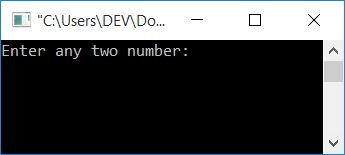Now supply any two numbers, say 10 and 20, then press the ENTER key to see the output as shown in the second snapshot of the same sample run: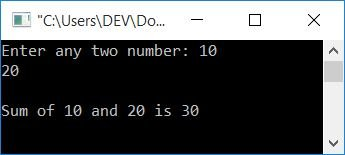#### Steps used in the above program

Here are some of the main steps involved in the above program:

• Declare any three variables, say num1, num2, and add.
• Here, num1 and num2 holds the first and second number entered by the user, and add will be used to store the addition result of the given two numbers.
• Scanning any two numbers entered by the user now.
• Print the value of add as output.

### Add two real numbers in C

The above program is only applicable for integer values. If the user enters any two numbers that contain a decimal, the above program will not be applicable. So here is the modified version of adding two numbers in C without regard to whether the entered number is an integer value or a real number.

```#include<stdio.h>
#include<conio.h>
int main()
{
printf("Enter any two number: ");
scanf("%f%f", &num1, &num2);
printf("\nSum of %0.2f and %0.2f is %0.2f", num1, num2, add);
getch();
return 0;
}```

The snapshot given here shows a sample run of the above program: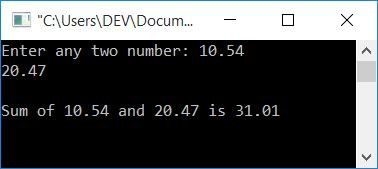As you can see from the above program, in place of int, declare all three variables of the float type.

Here, %0.2f is used to print a real number up to the second decimal place only.

Now let's create another program that does the same job, but this time using functions. Let's take a look at the program:

```#include<stdio.h>
#include<conio.h>
int sum(int, int);
int main()
{
printf("Enter any two number: ");
scanf("%d%d", &num1, &num2);
getch();
return 0;
}
int sum(int a, int b)
{
return a+b;
}```

Here is the sample run: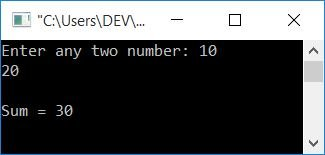## Add two numbers in C using recursion

The question is: write a program in C to add two numbers using a recursive function. Let's take a look at the program first:

```#include<stdio.h>
#include<conio.h>
int sum(int, int);
int main()
{
printf("Enter any two number: ");
scanf("%d%d", &num1, &num2);
getch();
return 0;
}
int sum(int a, int b)
{
if(b==0)
return a;
else
return (1+sum(a, b-1));
}```

Here is a screenshot of the above program's sample run: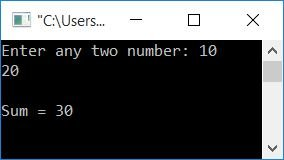#### The same program in different languages

C Quiz

« Previous Program Next Program »